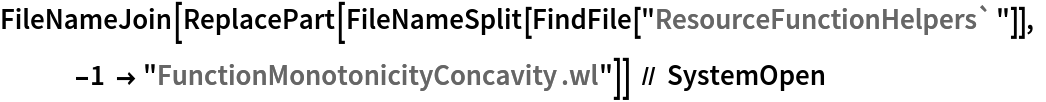Function Repository Resource:

# StrictlyMonotonicFunctionQ

Check if a function is strictly monotonic

Contributed by: Wolfram|Alpha Math Team
 ResourceFunction["StrictlyMonotonicFunctionQ"][f,x] gives True if f is strictly monotonic with respect to x. ResourceFunction["StrictlyMonotonicFunctionQ"][f,x,property] restricts the test to the direction dir.

## Details and Options

The direction dir can be any of Automatic, "Increasing" or "Decreasing", and defaults to Automatic. When dir is Automatic, ResourceFunction["StrictlyMonotonicFunctionQ"] returns True if f is strictly increasing or strictly decreasing.
ResourceFunction["StrictlyMonotonicFunctionQ"] expects f to be a univariate expression in terms of x, similar to what might be used in Plot.
ResourceFunction["StrictlyMonotonicFunctionQ"] returns True based on the definition of f being strictly monotonic; that is, f is strictly increasing on an interval A if for all {x,y}A such that x<y, it is the case that f[x]<f[y].

## Examples

### Basic Examples (2)

Compute whether an expression is strictly monotonic:

 In:=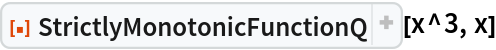Out=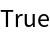Determine whether the expression is increasing:

 In:=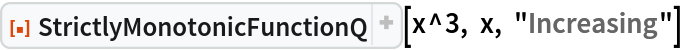Out=### Properties and Relations (3)

Note that a function with regions where it is constant is not strictly increasing:

 In:=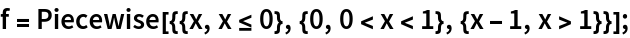In:=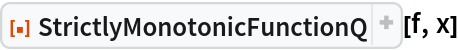Out=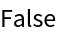For more information on the monotonicity of the input expression, use the resource function FunctionMonotonicity:

 In:=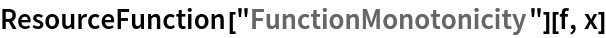Out=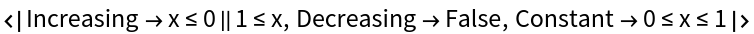Such a curve is only monotonic, not strictly monotonic:

 In:=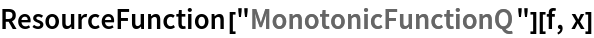Out=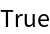## Publisher

Wolfram|Alpha Math Team

## Version History

• 2.0.0 – 23 March 2023
• 1.0.0 – 29 September 2020

## Author Notes

To view the full source code for StrictlyMonotonicFunctionQ, run the following code:

 In:=